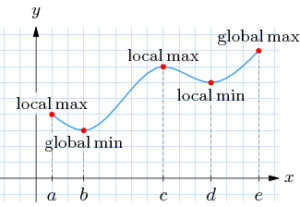# Global Maximum (Absolute Maximum): Definition

The global maximum of a function, or the extremum, is the largest value of the function. While there can be more than one local maximum in a function, there can be only one global maximum.

You can sometimes spot the location of the global maximum by looking at the graph of the whole function. For example:It makes sense the global maximum is located at the highest point. Similarly, the global minimum is located at the lowest point. The local maximum and minimum are the lowest values of a function given a certain range

Notice that in the graph above there are two endpoints, one located at x = a and one at x = e. These tell us that we are working with a function with a closed interval. Most of the questions you will encounter will ask you to find the global maximum of a function with a closed interval.

The other three points, b, c, and d are stationary points.  A stationary point is a point on the graph where the derivative is zero.The global maximum will always be located at one of the endpoints or at one of the high peaks of a stationary point. Therefore, we can limit our search for the global maximum to several points. Let’s take a look at an example.

## Example – Finding the Global Maximum

Find the global maximum of the following function:

f(x) = 1 + 2x3 – x4 [-1, 2]

The endpoints are given as [-1, 2]. That tells you that we will need to look at two function values: f(-1) and f(2).

Step 1: Find the first derivative of the function.

f′(x) = 6x2 – 4x3 = 0

Step 2: Set the first derivative to zero.

f′(x) = 2x2 (3 – 2x) = 0

Step 3: Look for stationary points. You’re going to make a logical leap or two here, using your knowledge of algebra.

For this particular equation, note that if you multiply 0 * (3 – 2x), you get zero, which meets the requirements of the equation. When does 2x2 equal 0?  When x is 0.  So x = 0 is our first stationary point.

(3 – 2x) will equal 0 when x is equal to 3/2.  So the second stationary point is x = 3/2.

Step 4: Calculate each value of y in order to locate the highest point.  Plugging each of our values of x into the function, we arrive at the following values for y:

• f(-1) = -2
• f(2) = 1 (i.e., 1 + 16 – 16 = 1)
• f(0) = 1
• f(3/2) = 2.688

Since the highest value is 2.688, that is the global maximum of the function.

## Advanced Methods for Finding the Global Maximum

Finding the global maximum for more complicated functions like the log-likelihood function sometimes involve complicated, multi-dimensional integrals. Several techniques have been formulated, including sampling the domain of the log-likelihood function and assessing the probability that relative maxima may or may not be global maxima. However, these tests are sensitive to model mismatch .

Another advanced method for finding global maximums (with constraints) is The Lagrange Multiplier.

## References

Blatt, D. On Tests for Global Maximum of the Log-Likelihood Function. Retrieved April 16, 2021 from: https://web.eecs.umich.edu/~hero/Preprints/LocalMaxTITver5.pdf

CITE THIS AS:
Stephanie Glen. "Global Maximum (Absolute Maximum): Definition" From StatisticsHowTo.com: Elementary Statistics for the rest of us! https://www.statisticshowto.com/global-maximum/